#Lambda Diagrams

Lambda Diagrams are a graphical notation for closed lambda terms, in which abstractions (lambdas) are represented by horizontal lines, variables by vertical lines emanating down from their binding lambda, and applications by horizontal links connecting the leftmost variables. In the alternative style, applications link the nearest deepest variables, for a more stylistic, if less uniform, look.

The following table shows diagrams of identity, the booleans, some standard combinators, some Church numerals, the predecessor function on Church numerals, and Omega.
 term definition diagram alternative I/1 λx.x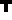K/true λx.λy.x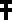false/0 λx.λy.y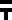S λx.λy.λz.(x z)(y z)Y λf.(λx.x x)(λx.f(x x))2 λf.λx.f(f x)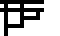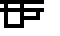3 λf.λx.f(f(f x))4 λf.λx.f(f(f(f x)))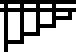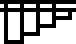pred λn.λf.λx.n(λg.λh.h(g f))(λu.x)(λu.u)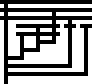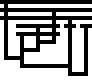fac λn.λf.n(λf.λn.n(f(λf.λx.n f(f x))))(λx.f)(λx.x)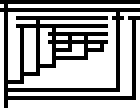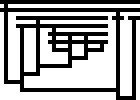fib λn.λf.n(λc.λa.λb.c b(λx.a (b x)))(λx.λy.x)(λx.x)f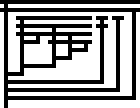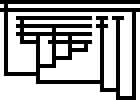Ω (λx.x x)(λx.x x)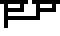Curiously, the alternative Omega diagram somewhat resembles an (upside-down) Omega.

And here, on a larger scale, is a prime number sieve (alternative style):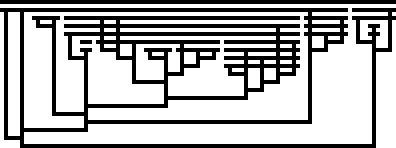which reduces to an infinite list of booleans that starts out as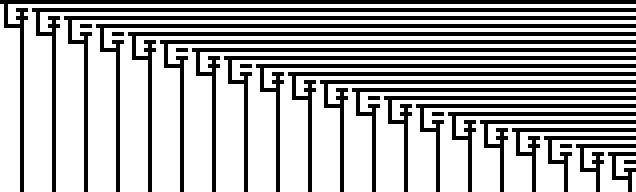## Dimensions and complexity

In terms of pixels, a diagram's width is one less than 4 times the number of variables (vertical lines), and its height is one more than twice the maximum number of nested abstractions and applications (one less for alternaitve diagrams with multiple variables).

The size of the binary encoding of a term is closely related to the graphical complexity: it is exactly twice the number of lines plus the number of (4-way) intersections.

## Reduction

Beta-reduction on lambda diagrams can be shown in several steps, as demonstrated in this reduction from Y=λf.(λx.x x)(λx.f(x x)) to λf.(λx.f(x x))(λx.f(x x))
 initial termshow application of abstractionshow bound variables and argument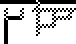expand function body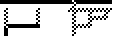to make room for substitutionsubstitute argument for variables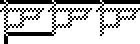final termIn the third frame, we show only the part of bound variables below abstractions, e.g. when applying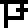, the function body x(λy.x) shows as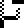.

## Diagrams In Motion

Paul Brauner has produced some awesome videos of beta reductions, produced with this software.

## Related work

Dave Keenan has a comprehensive online paper To Dissect a Mockingbird: A Graphical Notation for the Lambda Calculus with Animated Reduction, which partly inspired this page.

In his Master thesis, Viktor Massalõgin discusses 4 existing graphical notations before introducing his own "bubble" notation. Figure 3 on page 10 shows 4 depictions of the fixpoint combinator (which differs from Y above in one beta reduction), while the bubble form is in Figure 5 on page 13.

### Note

The diagram in the title, produced by the postscript code below, is a slightly deformed alternative Y diagram made to look like a Y.
```%!PS-Adobe-2.0 EPSF-2.0
%%BoundingBox:0 0 118 110
/m{moveto}def/l{lineto}def/c{concat 6 m 0 6 l 7 8 m 0 8 l l l 3 6 l 2 6 m 7 6 l
3 4 m 6 4 l 6 6 l stroke}def 3 0 0 0 1[-8 4 0 8 60 9]c 3 2 0 2 2[-1 1 0 1 0 0]c
```

Back to my Binary Lambda Calculus page.